# Counting How Many Times Specific Character Appears in Cell in Excel 2010

In this article we will learn counting how many times specific character appears in excel 2010.

To return the count of specific character in a cell we need to use a combination ofthe “LEN”, “TRIM”, and “SUBSTITUTE” functions..

LEN:Returns the number of characters in a text string.

Syntax of “LEN” function: =LEN (text)

Example: Cell A2 contains the text “By hook or by crook”
=LEN (“By hook or by crook”) will return 19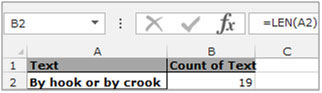TRIM:Trim function is used to remove additional spaces from the text in a cell.

Syntax of “TRIM” function: =TRIM (text)

Example: Cell A2 contains the text “By hook or by crook”
=TRIM (“By hook or by crook”) will return “By hook or by crook” without any extra leading and trailing spaces.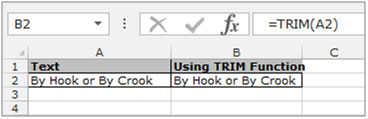SUBSTITUTE:This function is used to replace the old text with new text.

Syntax of “SUBSTITUTE” function:
=SUBSTITUTE (text,old_text,new_text,[instance_num])

Example: Cell A2 contains the text “By hook by crook”
=SUBSTITUTE (A2, A2,"By hook or by crook")
will return “By hook or by crook”.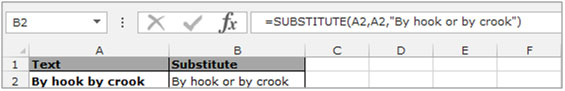Let’s take an example & understand how we can count a specific text in a cell:-

We have a text in cell A2, and we want to count the specific word characters “e” from the text. Follow the below given steps:-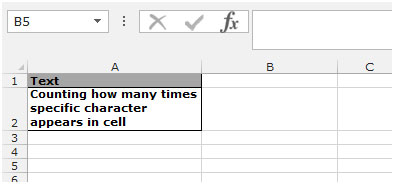• Select the cell B2 and write this formula.
• =IF(LEN(TRIM(A2))=0,0,LEN(TRIM(A2))-LEN(SUBSTITUTE(A2,"e",""))) and press Enter on the keyboard.
• The function will return 5, which means the character “e” is repeated 5 times in the cell A2.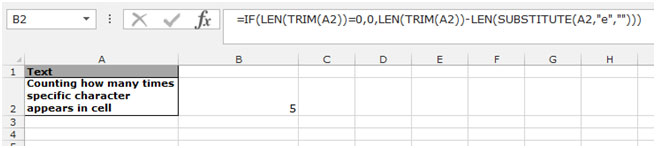1.Someone from the Internet

For it to work perfectly, you need to also wrap the last "A2" with TRIM as in the example below, I had issues with leading and trailing spaces, this way it was more reliable.
=IF(LEN(TRIM(A2))=0,0,LEN(TRIM(A2))-LEN(SUBSTITUTE(TRIM(A2),"e","")))

•This is pretty good idea.

2.Nice Tip.. Thanks for sharing 🙂

3.Wonderful. You could simple state that the idea is to find, how much shorter the string becomes, if you replace a specific character with "". If searching for the count of multi-character string within text, then have to divide by the length of hte sting.

But why is trimming necessary?

4.Your tip really helped me out. I wanted to the number of items in a cell that were separated by a comma. If there was only one item, I wanted a blank. I enclosed your tip and got the answers I wanted. with the 'comma' in H2 I wrote:
'=IF(LEN(G4)-LEN(SUBSTITUTE(G4,H\$2,""))=0,"",LEN(G4)-LEN(SUBSTITUTE(G4,H\$2,""))+1)

•=(LEN(A1)-LEN(SUBSTITUTE(UPPER(A1),UPPER(B1),"")))/LEN(B1)
Where A1 is the cell to calculate
B1 will be holding the character to be counted from A1

substitute formula returns the the new text instead of old text.

5.Great tip, very clever 🙂

•Thanks Rasmus:)

•Thanks for showing this! I do have one question though: it seems that only a single cell can be counted this way; when I tried to replace the single cell with a range of cells, the equation kept outputting "0". Is there a way to adapt this to counting the number of times a character appears, but in a range of cells, such as "C:C"?

Thank you!

6.This is the tip I was looking for years. Now, it becomes very easy to count characters. Great tip!!!

7.This is the tip I was looking for years. Now, it becomes very easy to count characters. Great tip!!!

Terms and Conditions of use

The applications/code on this site are distributed as is and without warranties or liability. In no event shall the owner of the copyrights, or the authors of the applications/code be liable for any loss of profit, any problems or any damage resulting from the use or evaluation of the applications/code.